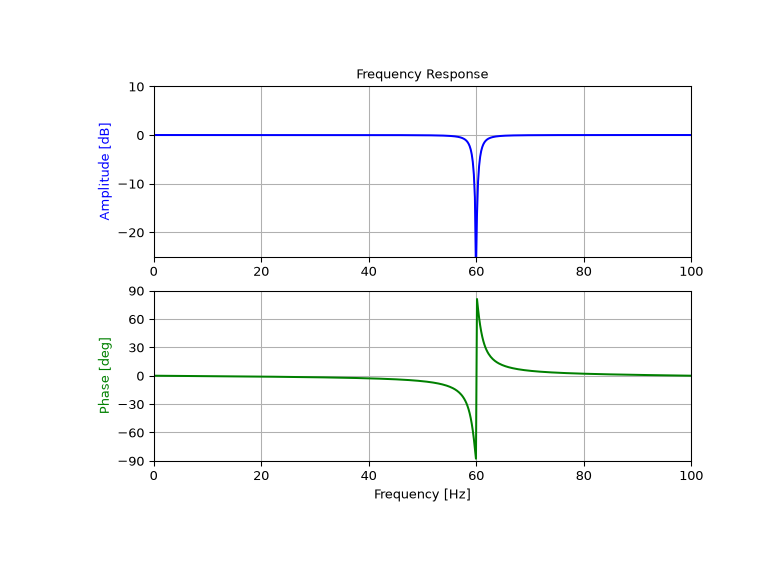# scipy.signal.iirnotch¶

scipy.signal.iirnotch(w0, Q, fs=2.0)[source]

Design second-order IIR notch digital filter.

A notch filter is a band-stop filter with a narrow bandwidth (high quality factor). It rejects a narrow frequency band and leaves the rest of the spectrum little changed.

Parameters
w0float

Frequency to remove from a signal. If fs is specified, this is in the same units as fs. By default, it is a normalized scalar that must satisfy `0 < w0 < 1`, with `w0 = 1` corresponding to half of the sampling frequency.

Qfloat

Quality factor. Dimensionless parameter that characterizes notch filter -3 dB bandwidth `bw` relative to its center frequency, `Q = w0/bw`.

fsfloat, optional

The sampling frequency of the digital system.

New in version 1.2.0.

Returns
b, andarray, ndarray

Numerator (`b`) and denominator (`a`) polynomials of the IIR filter.

Notes

New in version 0.19.0.

References

1

Sophocles J. Orfanidis, “Introduction To Signal Processing”, Prentice-Hall, 1996

Examples

Design and plot filter to remove the 60 Hz component from a signal sampled at 200 Hz, using a quality factor Q = 30

```>>> from scipy import signal
>>> import matplotlib.pyplot as plt
```
```>>> fs = 200.0  # Sample frequency (Hz)
>>> f0 = 60.0  # Frequency to be removed from signal (Hz)
>>> Q = 30.0  # Quality factor
>>> # Design notch filter
>>> b, a = signal.iirnotch(f0, Q, fs)
```
```>>> # Frequency response
>>> freq, h = signal.freqz(b, a, fs=fs)
>>> # Plot
>>> fig, ax = plt.subplots(2, 1, figsize=(8, 6))
>>> ax.plot(freq, 20*np.log10(abs(h)), color='blue')
>>> ax.set_title("Frequency Response")
>>> ax.set_ylabel("Amplitude (dB)", color='blue')
>>> ax.set_xlim([0, 100])
>>> ax.set_ylim([-25, 10])
>>> ax.grid()
>>> ax.plot(freq, np.unwrap(np.angle(h))*180/np.pi, color='green')
>>> ax.set_ylabel("Angle (degrees)", color='green')
>>> ax.set_xlabel("Frequency (Hz)")
>>> ax.set_xlim([0, 100])
>>> ax.set_yticks([-90, -60, -30, 0, 30, 60, 90])
>>> ax.set_ylim([-90, 90])
>>> ax.grid()
>>> plt.show()
```